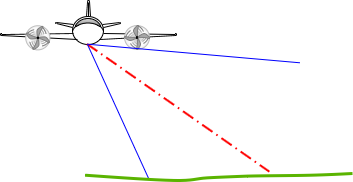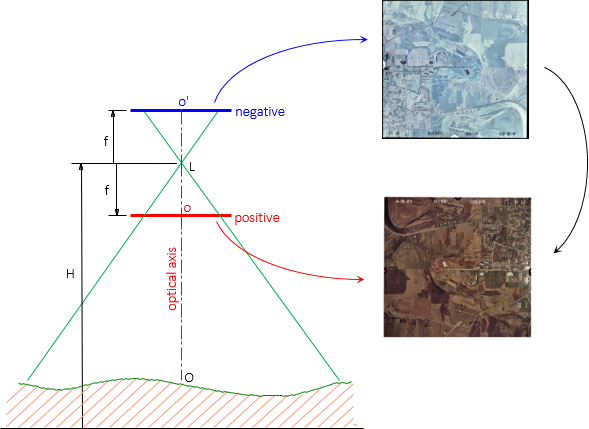## 1. Vertical Photography

Most metric mapping photographs are vertical, where the camera's optical axis is within 2° of vertical. Images for interpretative and/or support purposes can be intentionally tilted: low- (does not contain horizon) or high- (contains horizon) oblique, Figure C-1.(a) Low oblique (b) High oblique Figure C-1Tilted Photos

Panoramic images can also be captured by rotating the lens from horizon-to-horizon perpendicular to the flight path.

The basic geometry of a vertical aerial image is shown in Figure C-2.Figure C-2Vertical Photograph

Where:

L is the optical center of the lens.
f is the focal length, the perpendicular distance from the lens center to the negative.
H is the height above datum.

The image is captured on the negative where color and optical geometry are reversed, Figure C-3.Figure C-3Negative

A positive is a reversal of the negative; it's the image of the area we expect to see.

The physical size of the negative depends on the film used, usually 9" x 9" nominally for mapping. The positive may be larger or smaller depending on how it is produced. For most applications, a positive is created by contact printing making it the same size as the negative.

Using the same format sizes, Figure C-4 shows the addition of a positive to the geometry.Figure C-4Positive